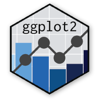Show Sidebar Hide Sidebar# facet_wrap in ggplot2

How to make subplots with facet_wrap in ggplot2 and R.

### New to Plotly?

Plotly's R library is free and open source!
You can set up Plotly to work in online or offline mode.
We also have a quick-reference cheatsheet (new!) to help you get started!

### Version Check

Version 4 of Plotly's R package is now available!
Check out this post for more information on breaking changes and new features available in this version.

library(plotly)
packageVersion('plotly')

##  '4.9.1'


### Basic Columns

library(reshape2)
library(plotly)

p <- ggplot(tips, aes(x=total_bill, y=tip/total_bill)) + geom_point(shape=1)

# Divide by day, going horizontally and wrapping with 2 columns
p <- p + facet_wrap( ~ day, ncol=2)

p <- ggplotly(p)

p


Inspired by Cookbook for R

library(plotly)

## read in data set (tolerance data from the ALDA book)
sep = ",", header = TRUE)

## change id and male to factor variables
tolerance <- within(tolerance, {
id <- factor(id)
male <- factor(male, levels = 0:1, labels = c("female", "male"))
})

p <- ggplot(data = tolerance, aes(x = time, y = tolerance)) + geom_point() +
stat_smooth(method = "lm", se = FALSE) + facet_wrap(~id)

p <- ggplotly(p)

p


Inspired by The IDRE at UCLA

library(plotly)

p <- ggplot(mpg, aes(displ, hwy))+
geom_point()+
stat_smooth()+
facet_wrap(~year)

p <- ggplotly(p)

p


Inspired by R Study Group

### Labels

library(plotly)
set.seed(123)

df <- diamonds[sample(1:nrow(diamonds), size = 1000), ]

# Create labels
labs <- c("Best","Second best","Third best","Average", "Average","Third Worst","Second Worst","Worst")
levels(df$clarity) <- rev(labs) p <- ggplot(df, aes(carat, price)) + geom_point() + facet_wrap(~ clarity) p <- ggplotly(p) p  Inspired by Stack Overflow ### Titles library(plotly) set.seed(123) df <- diamonds[sample(1:nrow(diamonds), size = 1000), ] # Create labels labs <- c("Best","Second best","Third best","Average", "Average","Third Worst","Second Worst","Worst") levels(df$clarity) <- rev(labs)

p <- ggplot(df, aes(carat, price)) +
geom_point() +
facet_wrap(~ clarity) +
ggtitle("Diamonds dataset facetted by clarity")

p <- ggplotly(p)

p


Inspired by ggplot2 Documentation

### Ordered Facets

library(plotly)
set.seed(123)

df <- diamonds[sample(1:nrow(diamonds), size = 1000), ]

# Reorer levels

levels(df\$clarity) <- c("VS2", "VS1", "VVS2", "I1", "SI2", "IF", "VVS1", "SI1")

p <- ggplot(df, aes(carat, price)) +
geom_point() +
facet_wrap(~ clarity) +
ggtitle("Diamonds dataset facetted by clarity")

p <- ggplotly(p)

p


Inspired by Stack Overflow